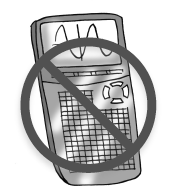### Home > GB8I > Chapter 10 Unit 11 > Lesson INT1: 10.1.3 > Problem10-46

10-46.

An auto repair shop is concerned about the reliability of its brake lathe. A lathe is a rotating piece of equipment used in restoring parts. The shop’s owner measured the speed (in rotations per minute) at various times over the course of a month. These were his measurements:$250$, $251$, $253$, $253$, $253$, $254$, $257$, $257$, $259$, $259$, $261$, $263$, $265$, $270$, $291$ rpm

1. On grid paper, draw a combination histogram and boxplot. Do not use a calculator.

Consider what an appropriate interval size would be for this data set.

1. Describe center, shape, spread, and outliers, similar to the way you did in problem 10-44.

Review the work you did on problem 10-44.

2. When reporting the center of this data, or the “typical rpm,” which would be more appropriate, the mean or the median? Why?

The median ($257$) is probably the more appropriate descriptor of the center.
This is because the outlier of $291$ has skewed the data some.
Removing the outlier causes the mean and the median to be nearly the same.